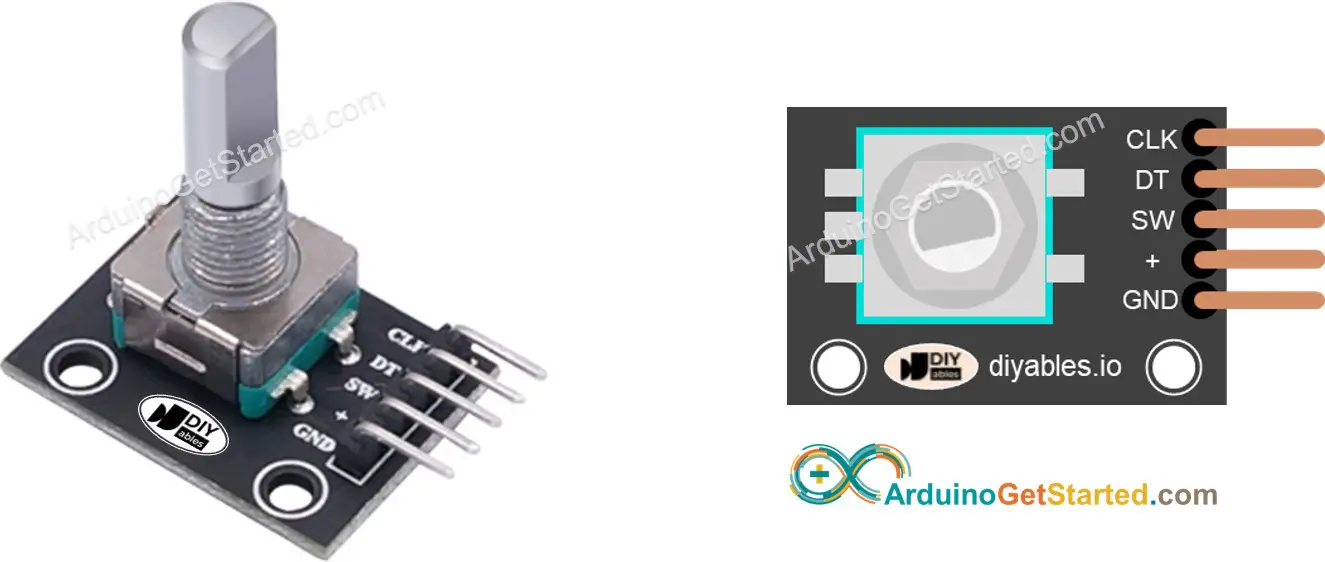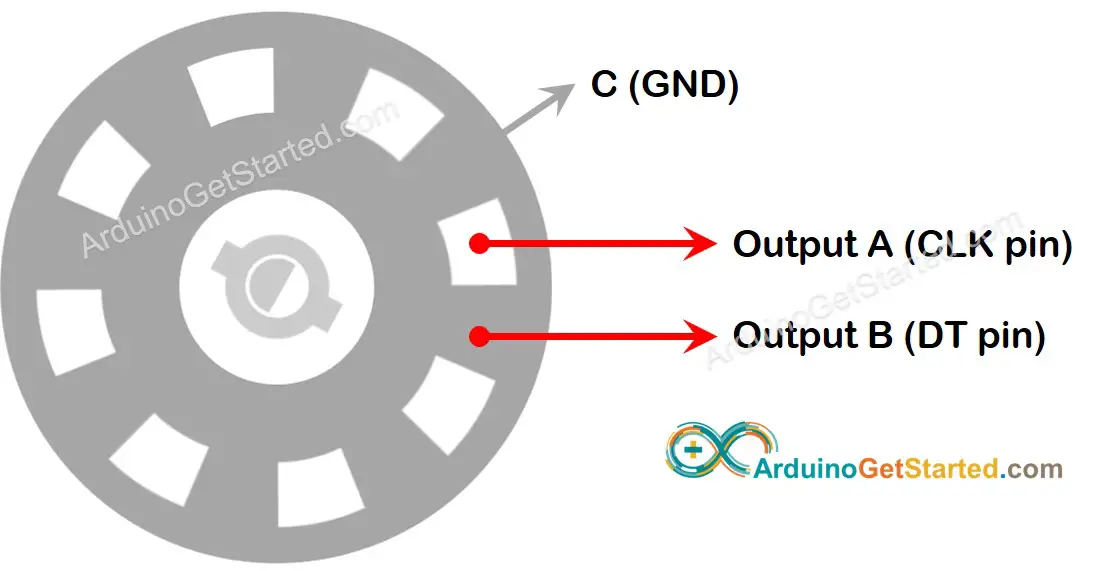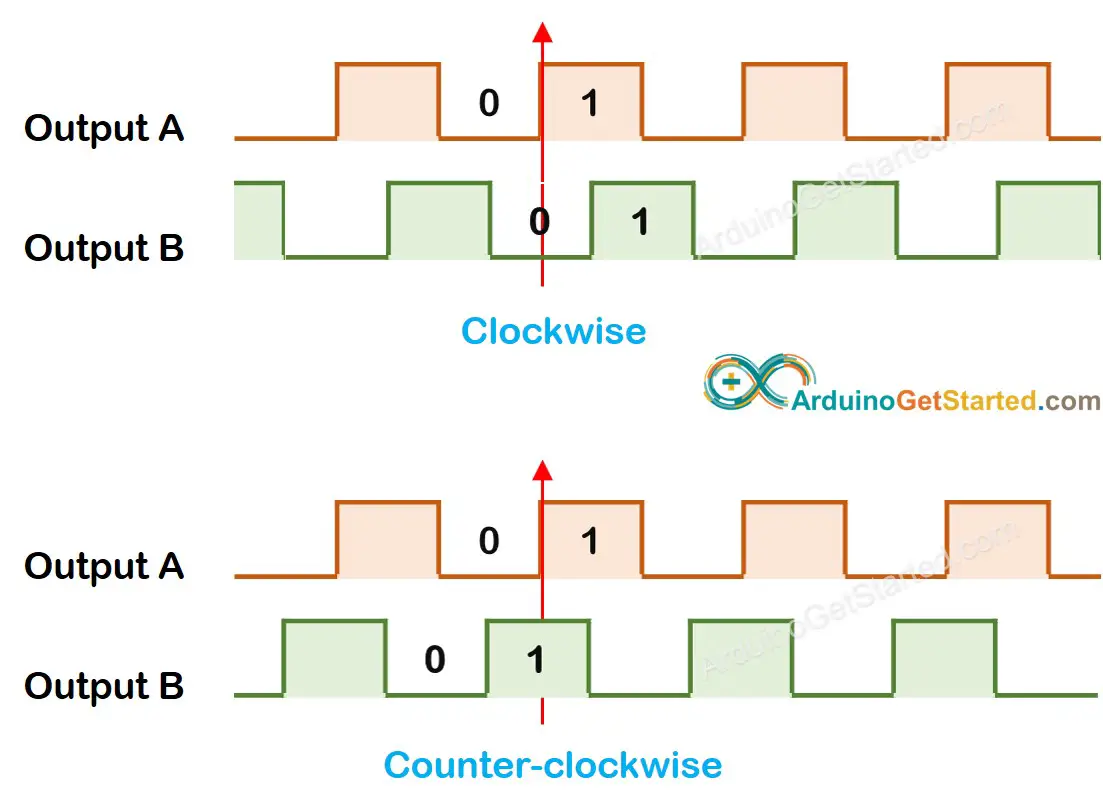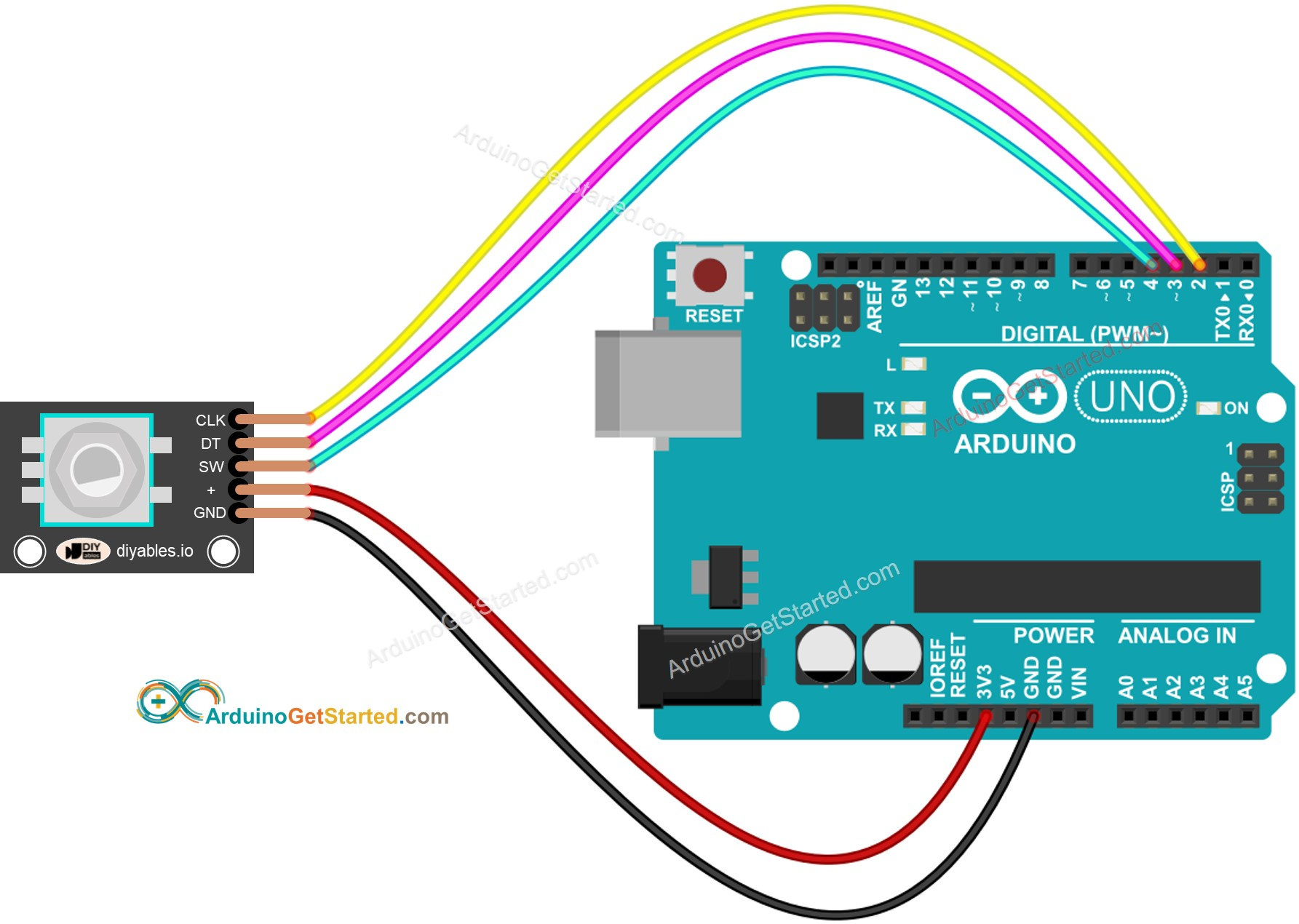# Arduino - Rotary Encoder

In this tutorial, we are going to learn how to use the incremental encoder with Arduino. In detail, we will learn:

• How a rotary encoder works
• Rotary encoder vs potentiometer
• How to connect the rotary encoder to Arduino
• How to program Arduino to read the direction and position from the rotary encoder WITHOUT interrupt
• How to program Arduino to read the direction and position from the rotary encoder WITH interrupt

## Hardware Required

Or you can buy the following sensor kit:

Please note: These are affiliate links. If you buy the components through these links, We may get a commission at no extra cost to you. We appreciate it.

A rotary encoder is an electromechanical device that converts rotational movement into an electrical signal. It measures the rotation and position of a shaft or knob. There are two main types:

• Incremental encoder: which generates pulses to measure relative change
• Absolute encoder: which provides a unique digital code for each position, making them ideal for precise positioning even after power loss.

This guide is about the incremental encoder.

### Rotary Encoder Module PinoutA rotary encoder module has 4 pins:

• CLK pin (Output A): is the main pulse that tells us how much rotation has occurred. Whenever you turn the knob by one detent (click) in either direction, the CLK pin outputs a signal that is a completes a full cycle (LOW HIGH LOW).
• DT pin (Output B): acts like the CLK pin but outputs a signal lags behind CLK signal by 90 degrees. It helps us figure out the direction of rotation (clockwise or anticlockwise).
• SW pin: is the output from the pushbutton inside the encoder. It’s normally open. If we use a pull-up resistor in this pin, the SW pin will be HIGH when the knob is not pressed, and LOW when it is pressed.
• VCC pin (+): needs to be connected to VCC (between 3.3 and 5 volts)
• GND pin: needs to be connected to GND (0V)

### Rotary Encoder vs Potentiometer

You may confuse the rotary encoder with the potentiometer. but they are distinct components. Here's a comparison between them:

• Rotary encoder is like the modern version of potentiometer, but they can do more things.
• Rotary encoder can spin around in a full circle without stopping, while potentiometer can only turn about three-quarters of the circle.
• Rotary encoder outputs pulses, while potentiometer outputs the analog voltage.
• Rotary encoder is handy when you just need to figure out how much the knob has moved, not exactly where it is. Potentiometer is useful when you really need to know exactly where a knob is.

### How Rotary Encoder WorksInside the encoder, there's a disc with slots connected to a pin called C, which is like a shared ground. There are two more pins, A and B.

• When you twist the knob, pins A and B touch the shared ground pin C, but in a certain order depending on which way you turn the knob (clockwise or counter-clockwise).
• These touches create two signals. They're a bit different in timing because one pin touches the ground before the other. Two signals are 90 degrees out of sync with each other. This is called quadrature encoding.
• When you turn the knob in clockwise direction, pin A touches the ground before pin B. When you turn the knob to the counterclockwise direction, pin B touches the ground before pin A.
• By monitoring when each pin touches or leaves the ground, we can figure out which way the knob is turning. We do this by checking what happens to pin B when pin A changes.When A changes states from LOW to HIGH:

• If B is LOW, the knob is turned clockwise.
• If B is HIGH, the knob is turned counter-clockwise.

※ NOTE THAT:

Pin A and B are connected to CLK and DT pins. However, depending on the manufacturers, the order may be different. The codes provided below are tested with the rotary encoder from DIYables

## How To Program For Rotary Encoder

• Check the signal from CLK pin
• If the state changes from LOW to HIGH, check the state of the DT pin.
• If the state of the DT pin is HIGH, the knob is turned in the counter-clockwise direction, increase the counter by 1
• If the state of the DT pin is LOW, the knob is turned in the clockwise direction, decrease the counter by 1

## Wiring DiagramThis image is created using Fritzing. Click to enlarge image

## Arduino Code – Rotary Encoder without Interrupt

The below Arduino code does:

• Detects the direction and amount of rotation of the encoder.
• If detecting the knob turned by one detent (click) in clockwise direction, increase the counter by one.
• If detecting the knob turned by one detent (click) in anticlockwise direction, decrease the counter by one.
• Detects if the button is pressed.
/* * Created by ArduinoGetStarted.com * * This example code is in the public domain * * Tutorial page: https://arduinogetstarted.com/tutorials/arduino-rotary-encoder */ #include <ezButton.h> // the library to use for SW pin #define CLK_PIN 2 #define DT_PIN 3 #define SW_PIN 4 #define DIRECTION_CW 0 // clockwise direction #define DIRECTION_CCW 1 // counter-clockwise direction int counter = 0; int direction = DIRECTION_CW; int CLK_state; int prev_CLK_state; ezButton button(SW_PIN); // create ezButton object that attach to pin 4 void setup() { Serial.begin(9600); // configure encoder pins as inputs pinMode(CLK_PIN, INPUT); pinMode(DT_PIN, INPUT); button.setDebounceTime(50); // set debounce time to 50 milliseconds // read the initial state of the rotary encoder's CLK pin prev_CLK_state = digitalRead(CLK_PIN); } void loop() { button.loop(); // MUST call the loop() function first // read the current state of the rotary encoder's CLK pin CLK_state = digitalRead(CLK_PIN); // If the state of CLK is changed, then pulse occurred // React to only the rising edge (from LOW to HIGH) to avoid double count if (CLK_state != prev_CLK_state && CLK_state == HIGH) { // if the DT state is HIGH // the encoder is rotating in counter-clockwise direction => decrease the counter if (digitalRead(DT_PIN) == HIGH) { counter--; direction = DIRECTION_CCW; } else { // the encoder is rotating in clockwise direction => increase the counter counter++; direction = DIRECTION_CW; } Serial.print("DIRECTION: "); if (direction == DIRECTION_CW) Serial.print("Clockwise"); else Serial.print("Counter-clockwise"); Serial.print(" | COUNTER: "); Serial.println(counter); } // save last CLK state prev_CLK_state = CLK_state; if (button.isPressed()) { Serial.println("The button is pressed"); } }

To simplify the code for button debouncing, the ezButton library is used.

#### Quick Steps

• Install ezButton library on Arduino IDE. See How To
• Copy the above code and open with Arduino IDE
• Click Upload button on Arduino IDE to upload code to Arduino
• Turn the knob in clockwise, then anticlockwise
• Press the knob
• See the result on Serial Monitor.
COM6
DIRECTION: Clockwise | COUNTER: 1 DIRECTION: Clockwise | COUNTER: 2 DIRECTION: Clockwise | COUNTER: 3 DIRECTION: Clockwise | COUNTER: 4 DIRECTION: Clockwise | COUNTER: 5 DIRECTION: Counter-clockwise | COUNTER: 4 DIRECTION: Counter-clockwise | COUNTER: 3 DIRECTION: Counter-clockwise | COUNTER: 2 DIRECTION: Counter-clockwise | COUNTER: 1 DIRECTION: Counter-clockwise | COUNTER: 0 The button is pressed
Autoscroll Show timestamp
Clear output
9600 baud
Newline

#### Code Explanation

Check out the line-by-line comments in the code

## Arduino Code – Rotary Encoder with Interrupt

In the previous example code, we use the polling method, which continuously check the pin's state. This has two disadvantages:

• Waste Arduino resource
• Some counter may be missed if another code takes long time to excecute.

One approach to handle this is by using interrupts. Interrupts eliminate the need for constant checking of a particular event. This allows the Arduino to carry out other tasks without overlooking an event.

Here’s an example of how to read a rotary encoder with interrupts.

/* * Created by ArduinoGetStarted.com * * This example code is in the public domain * * Tutorial page: https://arduinogetstarted.com/tutorials/arduino-rotary-encoder */ #include <ezButton.h> // the library to use for SW pin #define CLK_PIN 2 #define DT_PIN 3 #define SW_PIN 4 #define DIRECTION_CW 0 // clockwise direction #define DIRECTION_CCW 1 // counter-clockwise direction volatile int counter = 0; volatile int direction = DIRECTION_CW; volatile unsigned long last_time; // for debouncing int prev_counter; ezButton button(SW_PIN); // create ezButton object that attach to pin 4 void setup() { Serial.begin(9600); // configure encoder pins as inputs pinMode(CLK_PIN, INPUT); pinMode(DT_PIN, INPUT); button.setDebounceTime(50); // set debounce time to 50 milliseconds // use interrupt for CLK pin is enough // call ISR_encoderChange() when CLK pin changes from LOW to HIGH attachInterrupt(digitalPinToInterrupt(CLK_PIN), ISR_encoderChange, RISING); } void loop() { button.loop(); // MUST call the loop() function first if (prev_counter != counter) { Serial.print("DIRECTION: "); if (direction == DIRECTION_CW) Serial.print("Clockwise"); else Serial.print("Counter-clockwise"); Serial.print(" | COUNTER: "); Serial.println(counter); prev_counter = counter; } if (button.isPressed()) { Serial.println("The button is pressed"); } // TO DO: your other work here } void ISR_encoderChange() { if ((millis() - last_time) < 50) // debounce time is 50ms return; if (digitalRead(DT_PIN) == HIGH) { // the encoder is rotating in counter-clockwise direction => decrease the counter counter--; direction = DIRECTION_CCW; } else { // the encoder is rotating in clockwise direction => increase the counter counter++; direction = DIRECTION_CW; } last_time = millis(); }

Now, As you twist the knob, you'll notice information appearing on the Serial Monitor, much like what you saw in the earlier code.

※ NOTE THAT:

• If you use the interrupt, you need to connect the encoder's CLK pin to an Arduino pin that can handle interrupts. But remember, not all Arduino pins can do this. For example, on the Arduino Uno, only pins 2 and 3 can work with interrupts.
• You might come across tutorials on other websites that use two interrupts for a single encoder, but this is unnecessary and wasteful. Just one interrupt is sufficient.
• It's important to use the volatile keyword for global variables used in the interrupt. Neglecting this could lead to unexpected issues.
• Keep the code within the interrupt as straightforward as you can. Avoid using Serial.print() or Serial.println() inside the interrupt.

## Arduino Rotary Encoder Application

With Rotary Encoder, we can do the following applications but not limit:

• Arduino - Rotary Encoder controls Position of Sevo Motor
• Arduino - Rotary Encoder controls Brightness of LED
• Arduino - Rotary Encoder controls Speed of Stepper Motor

## Video Tutorial

We are considering to make the video tutorials. If you think the video tutorials are essential, please subscribe to our YouTube channel to give us motivation for making the videos.